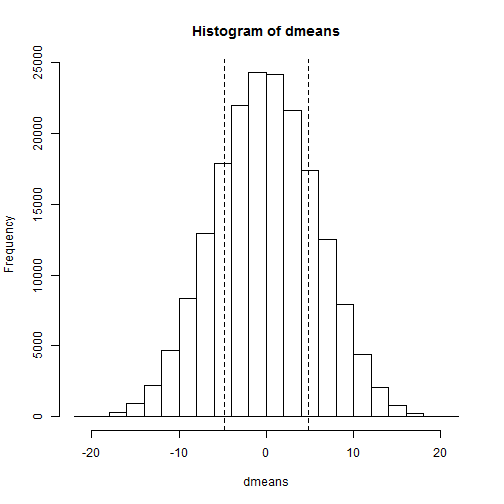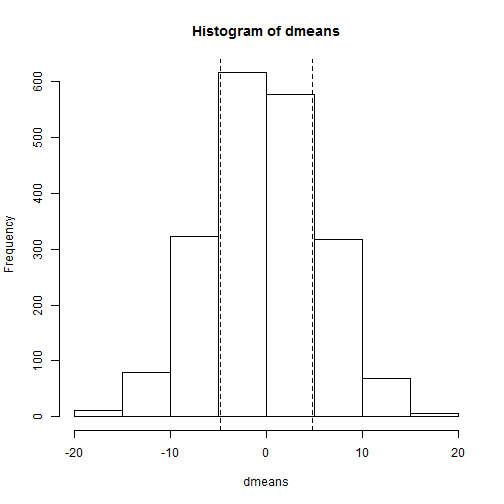# Permutation Tests

Let's talk about permutation tests and why we might want to do them.

First think about the two-sample t-test. The null hypothesis of this test is that both samples come from the same distribution. If we assume both samples come from the same approximately normal distribution, we can use math formulas based on probability theory to calculate a test statistic. We can then calculate the probability of getting such a test statistic (or one greater) under the assumption of both samples coming from the same distribution. For example, let's draw two samples from the same normal distribution and run a t-test.

set.seed(135)
s1 <- round(rnorm(10, 100, 16))
s2 <- round(rnorm(10, 100, 16))
t.test(s1, s2, var.equal = TRUE)

##
##  Two Sample t-test
##
## data:  s1 and s2
## t = 0.8193, df = 18, p-value = 0.4233
## alternative hypothesis: true difference in means is not equal to 0
## 95 percent confidence interval:
##  -7.508 17.108
## sample estimates:
## mean of x mean of y
##     102.4      97.6


As expected the p-value is high, telling us the difference in their means is not significant. Another interpretation is that the resulting t-test statistic (0.8193) would be likely if both samples came from the same distribution.

In real life we don't know with certainty the distribution of the population from which our samples are drawn. We also don't know the variance of the distribution or whether the distribution is even symmetric. We also may be stuck with a small sample size. Fortunately the t-test is pretty robust and usually reliable even when its assumptions are wrong. However, if you have your doubts, you can try a permutation test.

In the case our two-sample example above, the permutation test takes all possible combinations of group membership and creates a permutation distribution. In other words, if we assume both samples came from the same population, a data point in group 1 is just as likely to appear in group 2. If we determine all possible permutations, we can calculate our statistic of interest for each permutation and create a distribution. We can then assess where our original statistic falls in this distribution. If it's in the tails then we have evidence that our two samples come from two different populations. Now if your sample sizes are bigger than say, 15, creating the permutations can get computationally expensive very fast. So this is probably not something you want to do unless you're dealing with small samples.

Here's how we can do a permutation test “by hand” in R with our fake data:

d0 <- mean(s1) - mean(s2)
alls <- c(s1, s2)  # combine into one vector
N <- length(alls)
n <- length(s1)
p <- combn(N, n)  # generate all combinations of n chosen from N
dmeans <- numeric(dim(p))  # vector to store means
for (i in 1:dim(p)) {
dmeans[i] <- mean(alls[p[, i]]) - mean(alls[-p[, i]])
}
# plot histogram of all possible difference in means with lines indicating
# our original difference
hist(dmeans)
abline(v = d0, lty = 2)
abline(v = -d0, lty = 2)The combn function generates a matrix of all possible index combinations of n chosen from N. I say “index” because combn doesn't return the values themselves but rather their index position in the original vector. Thus we can use it to select all possible combinations from our combined vector, which is what we do in the for loop. In this case there are 184756 possible combinations. Once all possible differences in means are calculated we can plot a histogram of the differences and draw some lines to indicate where our original sample falls. Here I drew two lines to indicate the absolute value of the difference, the equivalent of a two-sided test. The probability (or p-value) of getting a difference more extreme than our original sample is pretty high. We can see this because there's a lot of histogram on either side of our lines. We can also calculate this value:

# two-sided p-value
signif((sum(dmeans <= -abs(d0)) + sum(dmeans >= abs(d0)))/dim(p))

##  0.4291


Very close to the p-value returned by the t-test.

Of course there are packages for this sort of thing. The one I know of is perm. Here's how we use its permTS function to replicate our results above:

library(perm)
permTS(s1, s2, alternative = "two.sided", method = "exact.ce",
control = permControl(tsmethod = "abs"))

##
##  Exact Permutation Test (complete enumeration)
##
## data:  s1 and s2
## p-value = 0.4291
## alternative hypothesis: true mean s1 - mean s2 is  0
## sample estimates:
## mean s1 - mean s2
##               4.8


The method= argument tells the function to do “complete enumeration”. The control= argument says how to calculate the p-value (i.e., the same way we did it “by hand”).

So that's a literal two-sample permutation test. As I mentioned, if your samples are large then this approach is not feasible as the number of permutations grows out of control. For example, two groups of size 20 results in 137,846,528,820 combinations. So we usually resort to resampling methods. This is where we repeatedly resample from our combined vector of values to get a large number of combinations. We don't generate all combinations, but enough to give us a good idea. Here's one way to do it:

dmeans <- numeric(2000)  # vector to store means
for (i in 1:2000) {
g <- sample(N, n)
dmeans[i] <- mean(alls[g]) - mean(alls[-g])
}
hist(dmeans)
abline(v = d0, lty = 2)
abline(v = -d0, lty = 2)signif((sum(dmeans <= -abs(d0)) + sum(dmeans >= abs(d0)))/2000)

##  0.4335


So out of 1.8476 × 105 possible permutations we only calculated 2000 (assuming no repeats) and we still got a p-value pretty close to that of our literal permutation test. Not bad.

We can do the same using the permTS function as follows:

permTS(s1, s2, alternative = "two.sided",
method = "exact.mc", control = permControl(nmc = 2000,
setSEED = FALSE, tsmethod = "abs"))

##
##  Exact Permutation Test Estimated by Monte Carlo
##
## data:  s1 and s2
## p-value = 0.4213
## alternative hypothesis: true mean s1 - mean s2 is  0
## sample estimates:
## mean s1 - mean s2
##               4.8
##
## p-value estimated from 2000 Monte Carlo replications
## 99 percent confidence interval on p-value:
##  0.3925 0.4498


The method “exact.mc” says use Monte Carlo simulation. The nmc= argument specifies number of replications (the default is 999). The setSEED= argument says not to set a seed. I want different random replications each time I run this line of code.

To wrap up, when does it make sense to use permutation tests? When you have something to permute! I quote from the classic text, An Introduction to the Bootstrap:

Permutation methods tend to apply to only a narrow range of problems. However when they apply, as in testing F = G in a two-sample problem, they give gratifyingly exact answers without parametric assumptions.

## 5 thoughts on “Permutation Tests”

1. John

Absolutely first class explanation.
Now trying to work out if I can do a similar thing for paired data…

2. prisma lop

Are you using a specific package to carry out the function:
dmeans <- numeric(dim(p))
for (i in 1:dim(p)) {
dmeans[i] <- mean(alls[p[, i]]) – mean(alls[-p[, i]])
}
hist(dmeans)
abline(v = d0, lty = 2)
abline(v = -d0, lty = 2)

This site uses Akismet to reduce spam. Learn how your comment data is processed.